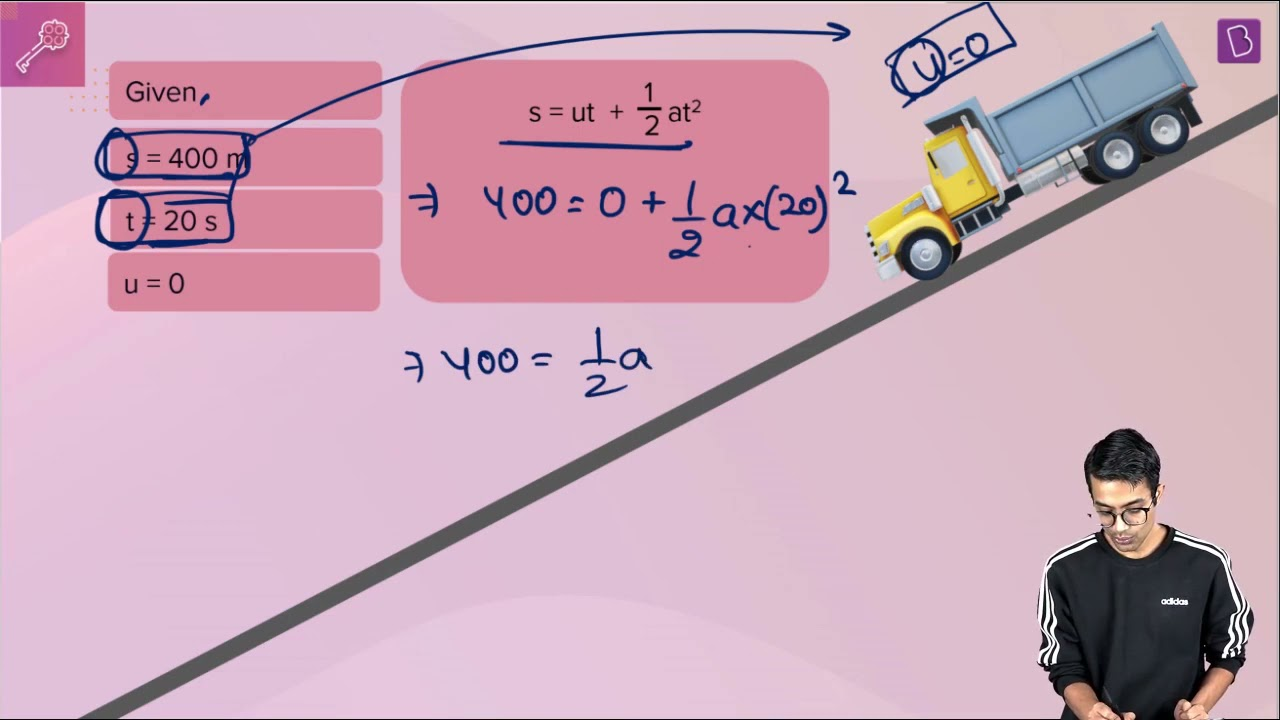# A truck starts from rest and rolls down a hill with a constant acceleration. It travels a distance of 400 m in 20 s. Find its acceleration. Find the force acting on it if its mass is 7 metric tons (Hint: 1 metric ton = 1000 kg).Given parameters

Initial velocity (u) = 0

Distance travelled (s) = 400 m

Time taken (t) = 20 s

Find out

The force acting on it if its mass is 7 metric tons

Solution

1 metric ton = 1000 kg

7 metric ton = 7 × 1000 = 7000 kg

∴ Mass (m) = 7000 kg

We know the formula

$$\begin{array}{l}s = ut + \frac{1}{2}at^{2}\end{array}$$

Substitute the given parameters in the above equation

400 = 0 + ½ a (20)2

400 = a × 400/2

400 = 200 × a

a = 2 m/s2

Acceleration is equal to 2 m/s2

Force (F) = Mass (m) × Acceleration (a)

Substitute the values of mass and acceleration in the above equation, we get

F = 7000 × 2

F = 14000 N

The force acting on it if its mass is 7 metric tons is 14000 N(504)(79)